•#### Mobile Artificial Intelligence Projects#### Overview of this book

We’re witnessing a revolution in Artificial Intelligence, thanks to breakthroughs in deep learning. Mobile Artificial Intelligence Projects empowers you to take part in this revolution by applying Artificial Intelligence (AI) techniques to design applications for natural language processing (NLP), robotics, and computer vision. This book teaches you to harness the power of AI in mobile applications along with learning the core functions of NLP, neural networks, deep learning, and mobile vision. It features a range of projects, covering tasks such as real-estate price prediction, recognizing hand-written digits, predicting car damage, and sentiment analysis. You will learn to utilize NLP and machine learning algorithms to make applications more predictive, proactive, and capable of making autonomous decisions with less human input. In the concluding chapters, you will work with popular libraries, such as TensorFlow Lite, CoreML, and PyTorch across Android and iOS platforms. By the end of this book, you will have developed exciting and more intuitive mobile applications that deliver a customized and more personalized experience to users.
PrefaceFree Chapter
Artificial Intelligence Concepts and FundamentalsCreating a Real-Estate Price Prediction Mobile AppImplementing Deep Net Architectures to Recognize Handwritten DigitsBuilding a Machine Vision Mobile App to Classify Flower SpeciesBuilding an ML Model to Predict Car Damage Using TensorFlowPyTorch Experiments on NLP and RNNTensorFlow on Mobile with Speech-to-Text with the WaveNet ModelImplementing GANs to Recognize Handwritten DigitsSentiment Analysis over Text Using LinearSVCWhat is Next?Other Books You May Enjoy# The mechanics behind ANNs

In this section, we will understand the nuts and bolts that are required to start building our own AI projects. We will get to grips with the common terms that are used in deep learning techniques.

This section aims to provide the essential theory at a high level, giving you enough insight so that you're able to build your own deep neural networks, tune them, and understand what it takes to make state-of-the-art neural networks.

# Biological neurons

We previously discussed how the biological brain has been an inspiration behind ANNs. The brain is made up of hundreds of billions of independent units or cells called neurons.

The following diagram depicts a neuron, and it has multiple inputs going into it, called dendrites. There is also an output going out of the cell body, called the axon: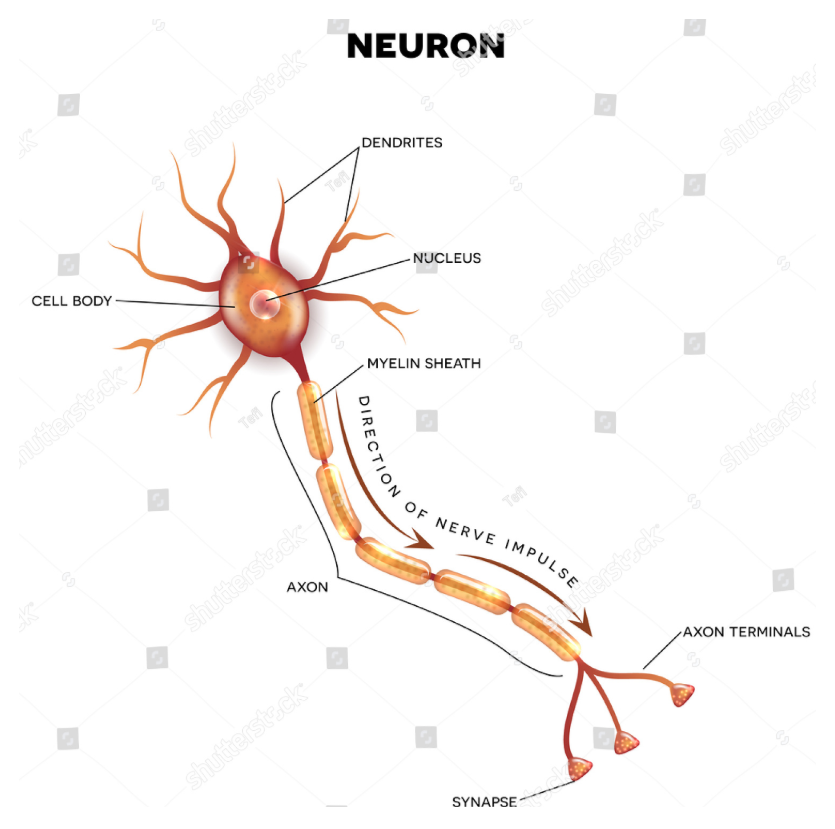The dendrites carry information into the neuron and the axon allows the processed information to flow out of the neuron. But in reality, there are thousands of dendrites feeding input into the neuron body as small electrical charges. If these small electrical charges that are carried by the dendrites have an effect on the overall charge of the body or cross over some threshold, then the axon will fire.

Now that we know how a biological neuron functions, we will understand how an artificial neuron works.

# Working of artificial neurons

Just like the biological brain, ANNs are made up of independent units called neurons. Like the biological neuron, the artificial neuron has a body that does some computation and has many inputs that are feeding into the cell body or neuron: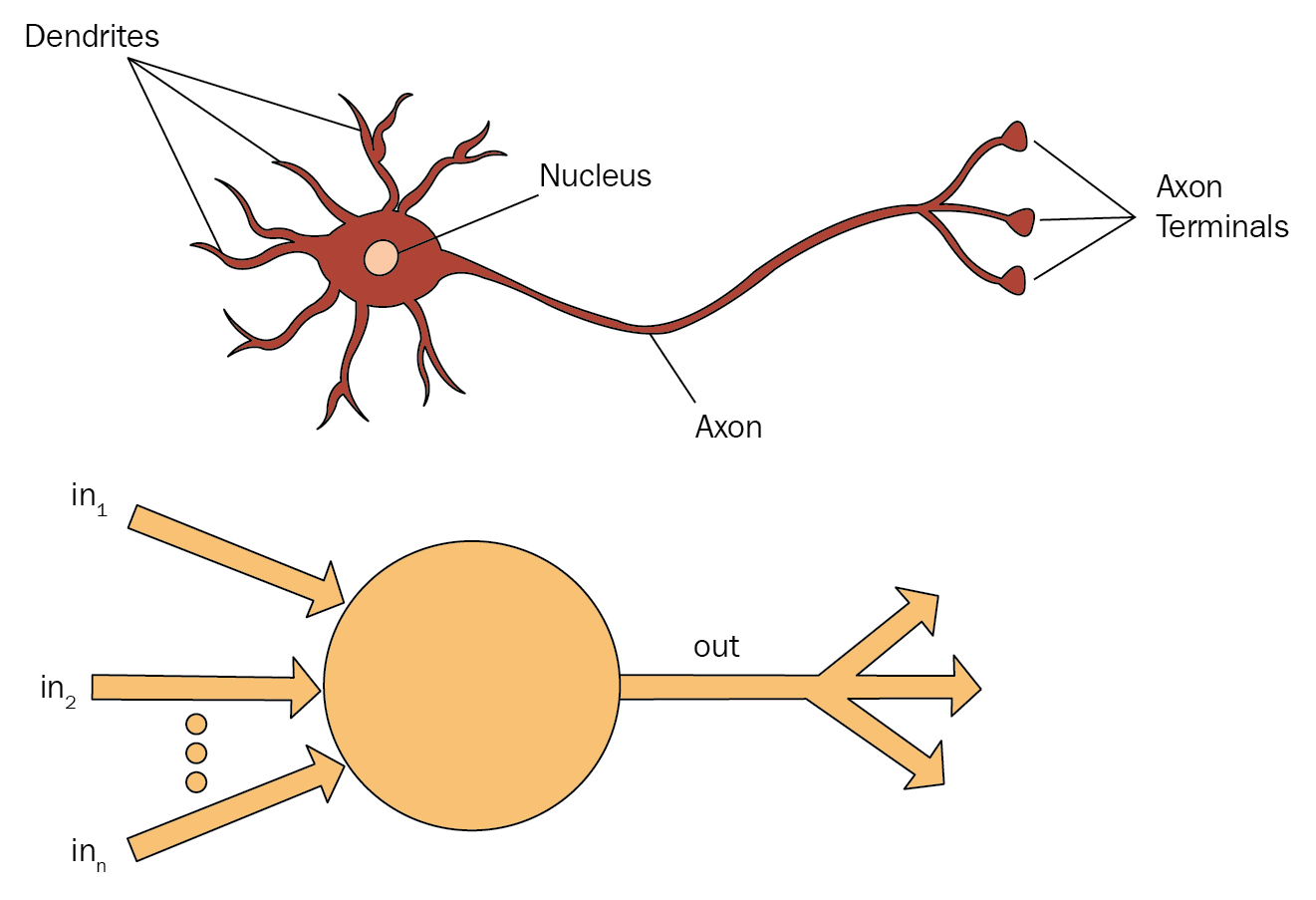For example, let's assume we have three inputs to the neuron. Each input carries a binary value of 0 or 1. We have an output flowing out of the body, which also carries a binary value of 0 or 1. For this example, the neuron decides whether I should eat a cake today or not. That is, the neuron should fire an output of 1 if I should eat a cake or fire 0 if I shouldn't:In our example, the three inputs represent the three factors that determine whether I should eat the cake or not. Each factor is given a weight of importance; for instance, the first factor is I did cardio yesterday and it has a weight of 2. The second factor is I went to the gym yesterday and weighs 3. The third factor is It is an occasion for cake and weighs 6.

The body of the neuron does some calculation to inputs, such as taking the sum of all of these inputs and checking whether it is over some threshold: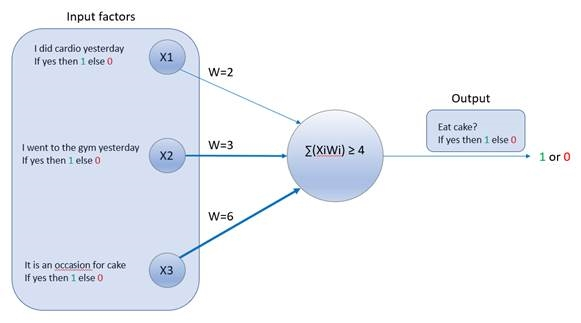So, for this example, let's set our threshold as 4. If the sum of the input weights is above the threshold, then the neuron fires an output of 1, indicating that I can eat the cake.

This can be expressed as an equation:Here, the following applies:

• Xi is the first input factor, I did cardio yesterday.
• Wi is the weight of the first input factor, Xi. In our example, Wi = 2.
• Xii is the second input factor, I went to the gym yesterday.
• Wii is the weight of the second input factor, Xii. In our example, Wii = 3.
• Xiii is the third input factor, It is an occasion for cake.
• Wiii is the weight of the third input factor, Xiii. In our example, Wiii = 6.
• threshold is 4.

Now, let's use this neuron to decide whether I can eat a cake for three different scenarios.

# Scenario 1

I want to eat a cake and I went to the gym yesterday, but I did not do cardio, nor is it an occasion for cake: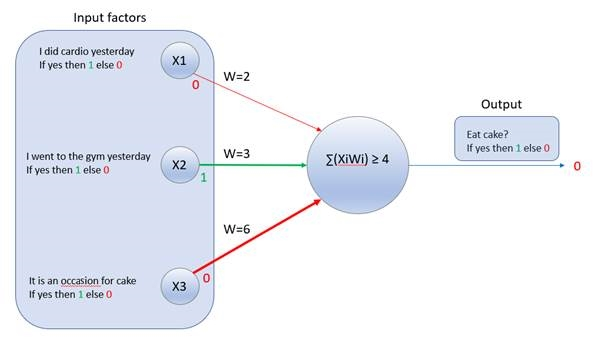Here, the following applies:

• Xi is the first input factor, I did cardio yesterday. Now, Xi = 0, as this is false.
• Wi is the weight of the first input factor, Xi. In our example, Wi = 2.
• Xii is the second input factor, I went to the gym yesterday. Now, Xii = 1, as this is true.
• Wii is the weight of the second input factor, Xii. In our example, Wii = 3.
• Xiii is the third input factor, It is an occasion for cake. Now, Xiii = 0, as this is false.
• Wiii is the weight of the third input factor, Xiii. In our example, Wiii = 6.
• threshold is 4.

We know that the neuron computes the following equation:For scenario 1, the equation will translate to this:This is equal to this:3 ≥ 4 is false, so it fires 0, which means I should not eat the cake.

# Scenario 2

I want to eat a cake and it's my birthday, but I did not do cardio, nor did I go to the gym yesterday: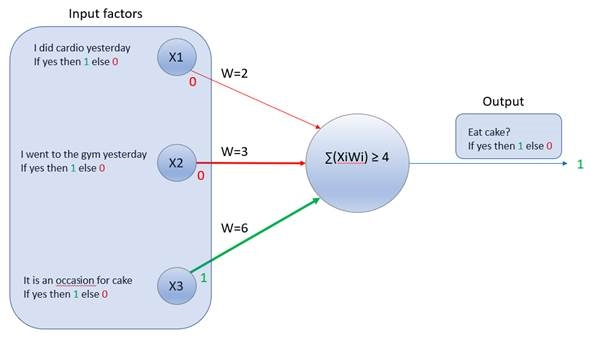Here, the following applies:

• Xi is the first input factor, I did cardio yesterday. Now, Xi = 0, as this factor is false.
• Wi is the weight of the first input factor, Xi. In our example, Wi = 2.
• Xii is the second input factor, I went to the gym yesterday. Now, Xii = 0, as this factor is false.
• Wii is the weight of the second input factor, Xii. In our example, Wii = 3.
• Xiii is the third input factor, It is an occasion for cake. Now, Xiii = 1, this factor is true.
• Wiii is the weight of the third input factor, Xiii. In our example, Wiii = 6.
• threshold is 4.

We know that the neuron computes the following equation:For scenario 2, the equation will translate to this:It gives us the following output:6 ≥ 4 is true, so this fires 1, which means I can eat the cake.

# Scenario 3

I want to eat a cake and I did cardio and went to the gym yesterday, but it is also not an occasion for cake: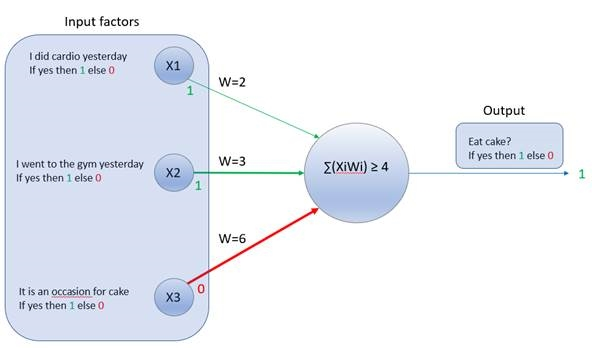Here, the following applies:

• Xi is the first input factor, I did cardio yesterday. Now, Xi = 1, as this factor is true.
• Wi is the weight of the first input factor, Xi. In our example, Wi = 2.
• Xii is the second input factor, I went to the gym yesterday. Now, Xii = 1, as this factor is true.
• Wii is the weight of the second input factor, Xii. In our example, Wii = 3.
• Xiii is the third input factor, It is an occasion for cake. Now, Xiii = 0, as this factor is false.
• Wiii is the weight of the third input factor, Xiii. In our example, Wiii = 6.
• threshold is 4.

We know that the neuron computes the following equation:For scenario 3, the equation will translate to this:This gives us the following equation:5 ≥ 4 is true, so this fires 1, which means I can eat the cake.

From the preceding three scenarios, we saw how a single artificial neuron works. This single unit is also called a perceptron. A perceptron essentially handles binary inputs, computes the sum, and then compares with a threshold to ultimately give a binary output.

To better appreciate how a perceptron works, we can translate our preceding equation into a more generalized form for the sake of explanation.

Let's assume there is just one input factor, for simplicity:Let's also assume that threshold = b. Our equation was as follows:It now becomes this:It can also be written as, then output 1 else 0.

Here, the following applies:

• w is the weight of the input
• b is the threshold and is referred to as the bias

This rule summarizes how a perceptron neuron works.

Just like the mammalian brain, an ANN is made up of many such perceptions that are stacked and layered together. In the next section, we will get an understanding of how these neurons work together within an ANN.

# ANNs

Like biological neurons, artificial neurons also do not exist on their own. They exist in a network with other neurons. Basically, the neurons exist by feeding information to each other; the outputs of some neurons are inputs to some other neurons.

In any ANN, the first layer is called the Input Layer. These inputs are real values, such as the factors with weights (w.x) in our previous example. The sum values from the input layer are propagated to each neuron in the next layer. The neurons of that layer do the computation and pass their output to the next layer, and so on: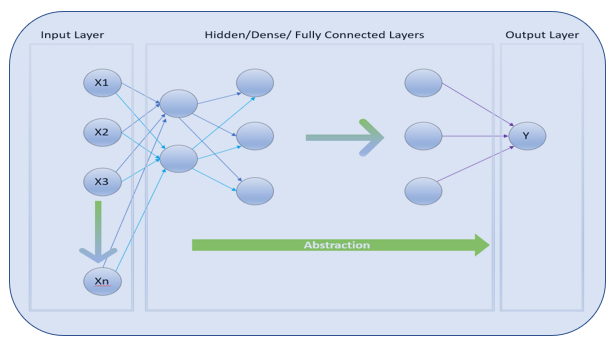The layer that receives input from all previous neurons and passes its output to all of the neurons of the next layer is called a Dense layer. As this layer is connected to all of the neurons of the previous and next layer, it is also commonly referred to as a Fully Connected Layer.

The input and computation flow from layer to layer and finally end at the Output Layer, which gives the end estimate of the whole ANN.

The layers in-between the input and the output layers are called the Hidden Layers, as the values of the neurons within these hidden layers are unknown and a complete black box to the practitioner.

As you increase the number of layers, you increase the abstraction of the network, which in turn increases the ability of the network to solve more complex problems. When there are over three hidden layers, then it is referred to as a deepnet.

So, if this was a machine vision task, then the first hidden layer would be looking for edges, the next would look for corners, the next for curves and simple shapes, and so on: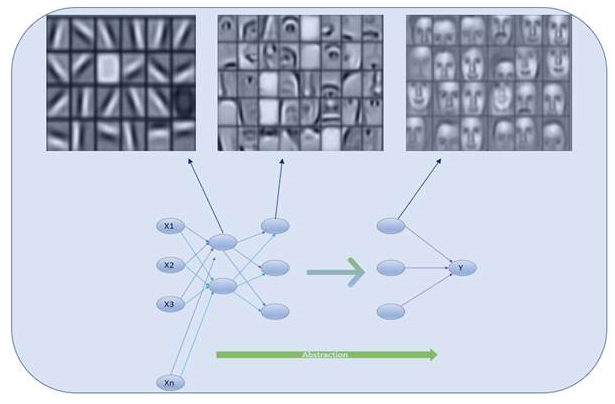Therefore, the complexity of the problem can determine the number of layers that are required; more layers lead to more abstractions. These layers can be very deep, with 1,000 or more layers, to very shallow, with just about half a dozen layers. Increasing the number of hidden layers does not necessarily give better results as the abstractions may be redundant.

So far, we have seen how artificial neurons can be stacked together to form a neural network. But we have seen that the perceptron neuron takes only binary input and gives only binary output. But in practice, there is a problem in doing things based on the perceptron's idea. This problem is addressed by activation functions.

# Activation functions

We now know that an ANN is created by stacking individual computing units called perceptrons. We have also seen how a perceptron works and have summarized it as Output 1, IF.

That is, it either outputs a 1 or a 0 depending on the values of the weight, w, and bias, b.

Let's look at the following diagram to understand why there is a problem with just outputting either a 1 or a 0. The following is a diagram of a simple perceptron with just a single input, x: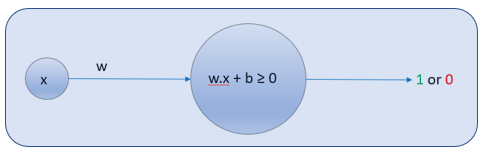For simplicity, let's call, where the following applies:

• w is the weight of the input, x, and b is the bias
• a is the output, which is either 1 or 0

Here, as the value of z changes, at some point, the output, a, changes from 0 to 1. As you can see, the change in output a is sudden and drastic: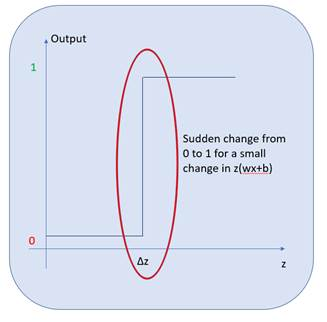What this means is that for some small change,, we get a dramatic change in the output, a. This is not particularly helpful if the perceptron is part of a network, because if each perceptron has such drastic change, it makes the network unstable and hence the network fails to learn.

Therefore, to make the network more efficient and stable, we need to slow down the way each perceptron learns. In other words, we need to eliminate this sudden change in output from 0 to 1 to a more gradual change: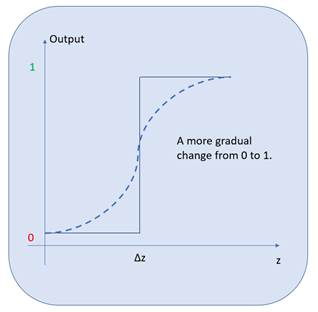This is made possible by activation functions. Activation functions are functions that are applied to a perceptron so that instead of outputting a 0 or a 1, it outputs any value between 0 and 1.

This means that each neuron can learn slower and at a greater level of detail by using smaller changes,. Activation functions can be looked at as transformation functions that are used to transform binary values in to a sequence of smaller values between a given minimum and maximum.

There are a number of ways to transform the binary outcomes to a sequence of values, namely the sigmoid function, the tanh function, and the ReLU function. We will have a quick look at each of these activation functions now.

# Sigmoid function

The sigmoid function is a function in mathematics that outputs a value between 0 and 1 for any input:Here,and.

Let's understand sigmoid functions better with the help of some simple code. If you do not have Python installed, no problem: we will use an online alternative for now at https://www.jdoodle.com/python-programming-online. We will go through a complete setup from scratch in Chapter 2, Creating a Real-Estate Price Prediction Mobile App. Right now, let's quickly continue with the online alternative.

Once we have the page at https://www.jdoodle.com/python-programming-online loaded, we can go through the code step by step and understand sigmoid functions:

1. First, let's import the math library so that we can use the exponential function:
`from   math   import  e`
1. Next, let's define a function called sigmoid, based on the earlier formula:
`def sigmoid ( x ):        return 1 / ( 1 + e **- x )`

1. Let's take a scenario where our z is very small, -10. Therefore, the function outputs a number that is very small and close to 0:
`sigmoid(-10) 4.539786870243442e-05`
1. If z is very large, such as 10000, then the function will output the maximum possible value, 1:
`sigmoid(10000)  1.0`

Therefore, the sigmoid function transforms any value, z, to a value between 0 and 1. When the sigmoid activation function is used on a neuron instead of the traditional perceptron algorithm, we get what is called a sigmoid neuron:

# Tanh function

Similar to the sigmoid neuron, we can apply an activation function called tanh(z), which transforms any value to a value between -1 and 1.

The neuron that uses this activation function is called a tanh neuron: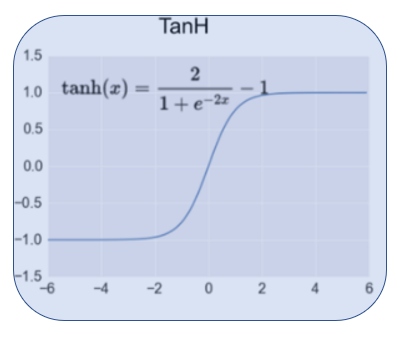# ReLU function

Then there is an activation function called the Rectified Linear Unit, ReLU(z), that transforms any value, z, to 0 or a value above 0. In other words, it outputs any value below 0 as 0 and any value above 0 as the value itself: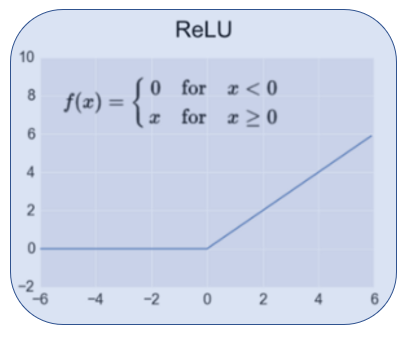Just to summarize our understanding so far, the perceptron is the traditional and outdated neuron that is rarely used in real implementations. They are great to get a simplistic understanding of the underlying principle; however, they had the problem of fast learning due to the drastic changes in output values.

We use activation functions to reduce the learning speed and determine finer changes in z or. Let's sum up these activation functions:

• The sigmoid neuron is the neuron that uses the sigmoid activation function to transform the output to a value between 0 and 1.
• The tanh neuron is the neuron that uses the tanh activation function to transform the output to a value between -1 and 1.
• The ReLU neuron is the neuron that uses the ReLU activation function to transform the output to a value of either 0 or any value above 0.

The sigmoid function is used in practice but is slow compared to the tanh and ReLU functions. The tanh and ReLU functions are commonly used activation functions. The ReLU function is also considered state of the art and is usually the first choice of activation function that's used to build ANNs.

Here is a list of commonly used activation functions: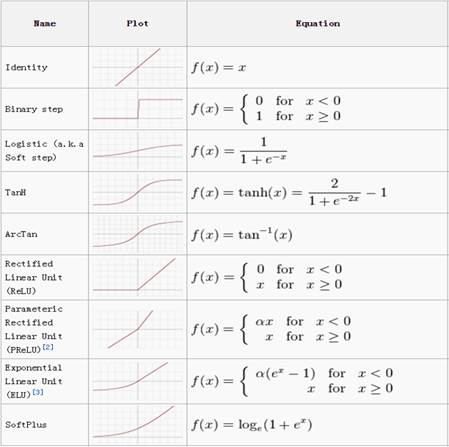In the projects within this book, we will be primarily using either the sigmoid, tanh, or the ReLU neurons to build our ANN.

# Cost functions

To quickly recap, we know how a basic perceptron works and its pitfalls. We then saw how activation functions overcame the perceptron's pitfalls, giving rise to other neuron types that are in use today.

Now, we are going to look at how we can tell when the neurons are wrong. For any type of neuron to learn, it needs to know when it outputs the wrong value and by what margin. The most common way to measure how wrong the neural network is, is to use a cost function.

A cost function quantifies the difference between the output we get from a neuron to an output that we need from that neuron. There are two common types of cost functions that are used: mean squared error and cross entropy.

# Mean squared error

The mean squared error (MSE) is also called a quadratic cost function as it uses the squared difference to measure the magnitude of the error:Here, the following applies:

• a is the output from the ANN
• y is the expected output
• n is the number of samples used

The cost function is pretty straightforward. For example, consider a single neuron with just one sample, (n=1). If the expected output is 2 (y=2) and the neuron outputs 3 (a=3), then the MSE is as follows:Similarly, if the expected output is 3 (y=3) and the neuron outputs 2 (a=2), then the MSE is as follows:Therefore, the MSE quantifies the magnitude of the error made by the neuron. One of the issues with MSE is that when the values in the network get large, the learning becomes slow. In other words, when the weights (w) and bias (b) or z get large, the learning becomes very slow. Keep in mind that we are talking about thousands of neurons in an ANN, which is why the learning slows down and eventually stagnates with no further learning.

# Cross entropy

Cross entropy is a derivative-based function as it uses the derivative of a specially designed equation, which is given as follows:Cross entropy allows the network to learn faster when the difference between the expected and actual output is greater. In other words, the bigger the error, the faster it helps the network learn. We will get our heads around this using some simple code.

Like before, for now, you can use an online alternative if you do not have Python already installed, at https://www.jdoodle.com/python-programming-online. We will cover the installation and setup in Chapter 2, Creating a Real-Estate Price Prediction Mobile App. Follow these steps to see how a network learns using cross entropy:

1. First, let's import the math library so that we can use the log function:
`from numpy import log  `
1. Next, let's define a function called cross_enrtopy, based on the preceding formula:
`def cross_entropy(y,a):     return -1 *(y*log(a)+(1-y)*log (1-a))`

1. For example, consider a single neuron with just one sample, (n=1). Say the expected output is 0 (y=0) and the neuron outputs 0.01 (a=0.01):
`cross_entropy(0, 0.01)`

The output is as follows:

`0.010050335853501451`

Since the expected and actual output values are very small, the resultant cost is very small.

Similarly, if the expected and actual output values are very large, then the resultant cost is still small:

`cross_entropy(1000,999.99) `

The output is as follows:

`0.010050335853501451`

Similarly, if the expected and actual output values are far apart, then the resultant cost is large:

`cross_entropy(0,0.9) `

The output is as follows:

`2.3025850929940459`

Therefore, the larger the difference in expected versus actual output, the faster the learning becomes. Using cross entropy, we can get the error of the network, and at the same time, the magnitude of the weights and bias is irrelevant, helping the network learn faster.

Up until now, we have covered the different kind of neurons based on the activation functions that are used. We have covered the ways to quantify inaccuracy in the output of a neuron using cost functions. Now, we need a mechanism to take that inaccuracy and remedy it.

The mechanism through which the network can learn to output values closer to the expected or desired output is called gradient descent. Gradient descent is a common approach in machine learning for finding the lowest cost possible.

To understand gradient descent, let's use the single neuron equation we have been using so far:Here, the following applies:

• x is the input
• w is the weight of the input
• b is the bias of the input

Gradient descent can be represented as follows: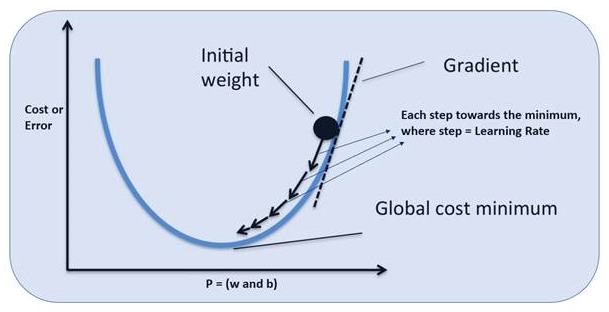Initially, the neuron starts by assigning random values for w and b. From that point onward, the neuron needs to adjust the values of w and b so that it lowers or decreases the error or cost (cross entropy).

Taking the derivative of the cross entropy (cost function) results in a step-by-step change in w and b in the direction of the lowest cost possible. In other words, gradient descent tries to find the finest line between the network output and expected output.

The weights are adjusted based on a parameter called the learning rate. The learning rate is the value that is adjusted to the weight of the neuron to get an output closer to the expected output.

Keep in mind that here, we have used only a single parameter; this is only to make things easier to comprehend. In reality, there are thousands upon millions of parameters that are taken into consideration to lower the cost.

# Backpropagation – a method for neural networks to learn

Great! We have come a long way, from looking at the biological neuron, to the types of neuron, to determining accuracy, and correcting the learning of the neuron. Only one question remains: how can the whole network of neurons learn together?

Backpropagation is an incredibly smart approach to making gradient descent happen throughout the network across all layers. Backpropagation leverages the chain rule from calculus to make it possible to transfer information back and forth through the network: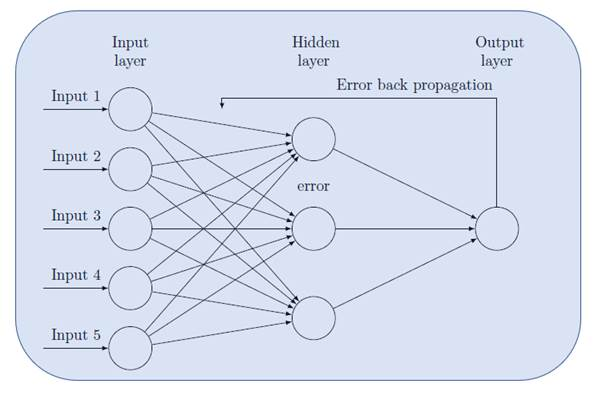In principle, the information from the input parameters and weights is propagated through the network to make a guess at the expected output and then the overall inaccuracy is backpropagated through the layers of the network so that the weights can be adjusted and the output can be guessed again.

This single cycle of learning is called a training step or iteration. Each iteration is performed on a batch of the input training samples. The number of samples in a batch is called batch size. When all of the input samples have been through an iteration or training step, then it is called an epoch.

For example, let's say there are 100 training samples and in every iteration or training step, there are 10 samples being used by the network to learn. Then, we can say that the batch size is 10 and it will take 10 iterations to complete a single epoch. Provided each batch has unique samples, that is, if every sample is used by the network at least once, then it is a single epoch.

This back-and-forth propagation of the predicted output and the cost through the network is how the network learns.

We will revisit training step, epoch, learning rate, cross entropy, batch size, and more during our hands-on sections.

# Softmax

We have reached our final conceptual topic for this chapter. We've covered types of neurons, cost functions, gradient descent, and finally a mechanism to apply gradient descent across the network, making it possible to learn over repeated iterations.

Previously, we saw the input layer and dense or hidden layers of an ANN: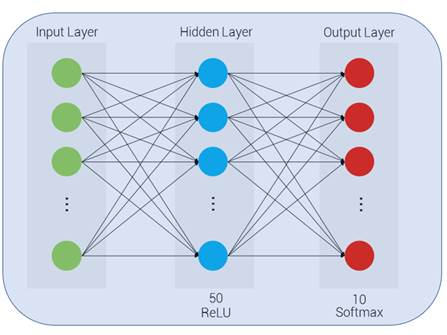Softmax is a special kind of neuron that's used in the output layer to describe the probability of the respective output:To understand the softmax equation and its concepts, we will be using some code. Like before, for now, you can use any online Python editor to follow the code.

First, import the exponential methods from the math library:

`     from math import exp `

For the sake of this example, let's say that this network is designed to classify three possible labels: A, B, and C. Let's say that there are three signals going into the softmax from the previous layers (-1, 1, 5):

`    a=[-1.0,1.0,5.0]`

The explanation is as follows:

• The first signal indicates that the output should be A, but is weak and is represented with a value of -1
• The second signal indicates that the output should be B and is slightly stronger and represented with a value of 1
• The third signal is the strongest, indicating that the output should be C and is represented with a value of 5

These represented values are confidence measures of what the expected output should be.

Now, let's take the numerator of the softmax for the first signal, guessing that the output is A:Here, M is the output signal strength indicating that the output should be A:

`exp(a) # taking the first element of a[-1,1,5] which represents A0.36787944117144233`

Next, there's the numerator of the softmax for the second signal, guessing that the output is B:Here, M is the output signal strength indicating that the output should be B:

`exp(a) # taking the second element of a[-1,1,5] which represents B2.718281828459045`

Finally, there's the numerator of the softmax for the second signal, guessing that the output is C:Here, M is the output signal strength indicating that the output should be C:

`exp(a) # taking the third element of a[-1,1,5] which represents C148.4131591025766`

We can observe that the represented confidence values are always placed above 0 and that the resultant is made exponentially larger.

Now, let's interpret the denominator of the softmax function, which is a sum of the exponential of each signal value:Let's write some code for softmax function:

`sigma = exp ( a [ 0 ]) + exp ( a [ 1 ]) + exp ( a [ 2 ]) sigma151.49932037220708`

Therefore, the probability that the first signal is correct is as follows:

`exp(a)/sigma0.0024282580295913376`

This is less than a 1% chance that it is A.

Similarly, the probability that the third signal is correct is as follows:

`exp(a)/sigma0.9796292071670795`

This means there is over a 97% chance that the expected output is indeed C.

Essentially, the softmax accepts a weighted signal that indicates the confidence of some class prediction and outputs a probability score between 0 to 1 for all of those classes.

Great! We have made it through the essential high-level theory that's required to get us hands on with our projects. Next up, we will summarize our understanding of these concepts by exploring the TensorFlow Playground.